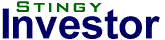Contact - Subscribe - LoginHome | Articles | Screens | Links | SNW | Rothery Report

 Overview Simple Screens High Dividend Yield   High Yield DJIA30   High Yield TSX60 Low P/E   Low P/E DJIA   Low P/E TSX60 Low P/B   Low P/B DJIA   Low P/B TSX60 Dividends at Risk   Dividend Risk DJIA30   Dividend Risk TSX60 Value Ratio   Value Ratio DJIA30   Value Ratio TSX60 + FAQ Tools:  Asset Mixer  Periodic Table
Value Screen Notes

The ratio columns display quintile scores and not the ratio itself. A 5 represents the 20% of stocks with the lowest ratios. A Score of 1 is given to the 20% of stocks with the highest ratios. Stocks with negative ratios are given a score of 0.

However, yield is the exception. It has the same quintile score as the P/D column. That is, high yield scores are given to stocks with high yields. Low yield scores are given to stocks with low yields. A 0 yield score is given to stocks that don't pay dividends.

Generally speaking, stocks with high quintile scores represent better values.

P/E = Price to Earnings Ratio Quintile
P/B = Price to Book Value Ratio Quintile
P/S = Price to Sales Ratio Quintile
P/C = Price to Cash Flow Ratio Quintile
P/D = Price to Dividend Ratio Quintile
Yield = Dividend Yield Quintile
G\$ = square root of (22.5 * EPS * BPS)
dG\$ = 100% * (G\$ - Price)/Price
VR = P/E Ratio divided by Dividend Yield

where

EPS = Earnings per share
BPS = Book Value per share

G\$ and dG\$

Benjamin Graham demanded that his defensive stocks have a combined P/E times P/B ratio of less than 22.5. The G\$ represents the price at which a stock would have a P/E times P/B equal to 22.5. As a result, a price less than the G\$ would represent a relatively good value.

The dG\$ is the percentage amount that a stock would have to rise by to hit the G\$. All else being equal, a higher dG\$ is better.

A word of caution

The value screens are provided for informational purposes only. They are not intended to represent recommendations or financial advice. Be sure to do your own due diligence. Consult with your investment advisor before making any changes to your portfolio.